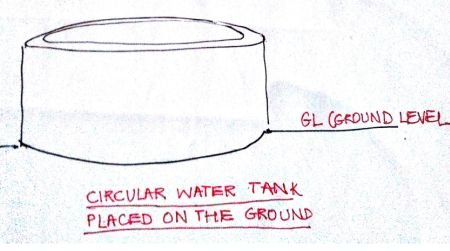## Design Procedure for Circular Water tank

#### In our previous article, we discussed some important theory and formulas required in the design procedure of Circular Water Tank.We will now move on with the stepwise design procedure for Water tank design.

#### Step One

Determine the design constants such as σcbc, σct, m, σst

Where,

σcbc = permissible compressive stress in concrete

σct = permissible tensile stress in concrete

m = modular ratio

σst = permissible compressive stress in steel

#### Step two

Determine:

1. Dimensions of the tank
2. Volume of the tank
3. Area of the tank (by assuming its depth)